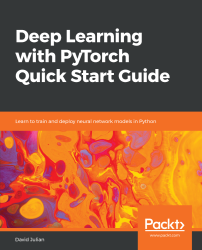•#### Deep Learning with PyTorch Quick Start Guide#### Overview of this book

PyTorch is extremely powerful and yet easy to learn. It provides advanced features, such as supporting multiprocessor, distributed, and parallel computation. This book is an excellent entry point for those wanting to explore deep learning with PyTorch to harness its power. This book will introduce you to the PyTorch deep learning library and teach you how to train deep learning models without any hassle. We will set up the deep learning environment using PyTorch, and then train and deploy different types of deep learning models, such as CNN, RNN, and autoencoders. You will learn how to optimize models by tuning hyperparameters and how to use PyTorch in multiprocessor and distributed environments. We will discuss long short-term memory network (LSTMs) and build a language model to predict text. By the end of this book, you will be familiar with PyTorch's capabilities and be able to utilize the library to train your neural networks with relative ease.
PrefaceFree Chapter
Introduction to PyTorchDeep Learning FundamentalsComputational Graphs and Linear ModelsConvolutional NetworksOther NN ArchitecturesGetting the Most out of PyTorchOther Books You May Enjoy# Other NN Architectures

Recurrent networks are essentially feedforward networks that retain state. All the networks we have looked at so far require an input of a fixed size, such as an image, and give a fixed size output, such as the probabilities of a particular class. Recurrent networks are different in that they accept a sequence, of arbitrary size, as the input and produce a sequence as output. Moreover, the internal state of the network's hidden layers is updated as a result of a learned function and the input. In this way, a recurrent network remembers its state. Subsequent states are a function of previous states.

In this chapter, we will cover the following:

• Introduction to recurrent networks
• Long short-term memory networks